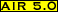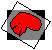# reslice_warp

## Purpose:

This program takes a .warp file and uses the information that it contains to load the corresponding image file and generate a new, realigned file.

## Usage:

reslice_warp .warp-file output [options]

options:
[-a alternate-reslice-file]
changes the file to be resliced
[-d divide-by-intensity-scale-factor]scales output by dividing by specified factor
[-df divide-by-intensity-scale-factor-file]scales output by dividing by factor specified in file
[-n model {half-window-width(s)}]
changes interpolation model
[-o]
grants permission to overwrite output
[-s multiplicative-intensity-scale-factor]
scales output using specified multiplicative factor
[-sf multiplicative-intensity-scale-factor-file]scales output using multiplicative factor specified in file
where the following definitions apply:
alternate-reslice-file [-a]
name of an alternate reslice file that is spatially equivalent (same voxel sizes and dimensions) to the default reslice file specified in the .warp-file
divide-by-intensity-scale-factor [-d]
output intensities are divided by this positive value
divide-by-intensity-scale-factor-file [-df]
output intensities are divided by the value in this ASCII file containing a single positive number
half-window-width [-n ...]
half the width of the window (in whole voxels) to apply when doing sinc interpolation
larger values are more accurate, but considerably slower
model [-n ...]
the interpolation model number, selected from the menu:
• 0. nearest neighbor
• 1. trilinear
• 2. windowed sinc in original xy plane, linear along z
• 3. windowed sinc in original xz plane. linear along y
• 4. windowed sinc in original yz plane, linear along x
• 5. 3D windowed sinc
multiplicative-intensity-scale-factor [-s]
output intensities are multiplied by this positive value
multiplicative-intensity-scale-factor-file [-sf]
output intensities are multiplied by the value in this ASCII file containing a single positive number
output [mandatory]
name of the output image file (.hdr or .img suffix optional)
.warp-file [mandatory]
name of the .warp file containing the nonlinear transformation parameters

## Examples:

reslice_warp mri.warpatlas newmri -o
• The reslice file identified in mri.warpatlas will be resampled based on the nonlinear spatial transformation parameters in mri.warpatlas. The newly created file will be called newmri.img and will overwrite any existing file with that name.

• Unlike reslice, this program does not interpolate output to create cubic voxels.
• Trilinear interpolation is the default interpolation method for this program.
• This program does not exclude regions where the resampling produces a local spatial inversion. The program determinant_mask can be used to create a mask that can be applied to the output of this program to remove such regions.
• If you have registered data using edited versions of files but then reslice unedited versions, you may see bizarre reduplications of data outside the brain. This is due to the fact that the nonlinear transformation can cause the reslice file to bend back on itself or create mirror image ghosts. In theory this can even happen within the brain, but you should not typically encounter this within the brain unless you have ignored advice about use of the program align_warp.
• The half window widths for sinc interpolation control the number of surrounding voxels that contribute to the interpolated value along each axis of the reslice file. A half-window width of 1 results in interpolation along that axis that only includes the nearest neighbor on either side and a half-window width of 6 will include the six nearest neighboring voxels in either direction. The total number of voxel included for standard 3D sinc interpolation is therefore 8*(x_half_window_width)*(y_half_window_width*(z_half_window_width). The larger the half windows,the more closely the interpolation will match true sinc interpolation, but also the slower the resampling process.
• Windowing of sinc interpolation is implemented using a Hanning window function exactly as described by Hajnal JV, Saeed N, Soar EJ, Oatridge A, Young IR, Bydder GM. Journal of Computer Assisted Tomography 1995:19:289-296.

## Error messages: (alphabetical by case)

-a must be followed by an image file name
• supply an image file name; this file must have the same dimensions and voxel sizes as the reslice file specified in the .warp file. The programs scan_warp and scanheader will allow you to verify this.
-d must be followed by a positive number
• supply a positive number
-df must be followed by a file name
• supply a file name; this must be a plain text ASCII file containing a single number
-n ____ does not define a valid interpolation model
• choose a model number from the menu
-n ____ must be followed by three positive integers
• certain sinc interpolation models require three half-window-widths
-n ____ must be followed by two positive integers
• certain sinc interpolation models require two half-window-widths
-n must be followed by a valid interpolation model number
• choose a model number from the menu
-s must be followed by a positive number
• supply a positive number
-sf must be followed by a file name
• supply a file name; this must be plain text ASCII file containing a single number
Unable to parse ____
• check syntax for undefined flags
Unable to parse argument ____, which was expected to begin with a '-'
• check syntax, an argument without a flag is positioned as if were a flagModified: December 15, 2001